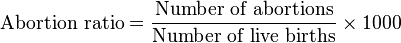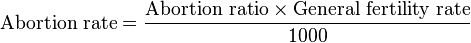Abortion ratio

This article describes a ratio measure. In the standard operationalization, the numerator of the measure is number of abortions (view other ratio measures with this numerator) and the denominator of the measure is number of live births (view other ratio measures with this denominator). The denominator used for reporting is 1000. The measurement period is a variable.
The numerator counts the number of occurrences of an event during the measurement period.
The denominator counts the number of occurrences of an event.

Definition

The abortion ratio for a population, typically measured in a given year (but with the measurement period variable in principle) is the number of abortions for every 1000 live births in the population. In other words:$\mbox{Abortion ratio} = \frac{\mbox{Number of abortions}}{\mbox{Number of live births}} \times 1000$

The abortion ratio could be any nonnegative real value, and in principle may be more than 1000 (indicating more abortions than live births). Such high abortion rates, though rare, have been recorded, such as in Russia during Communist rule.

Note that the abortion ratio does not take into account the pregnancies that result in spontaneous fetal death or stillbirth, either in the numerator or in the denominator.

Relation with abortion rate

The abortion ratio is related to but distinct from the abortion rate. The abortion rate has denominator the total number of women of childbearing age, rather than the total number of live births. They are related via the general fertility rate. Explicitly:$\mbox{Abortion rate} = \frac{\mbox{Abortion ratio} \times \mbox{General fertility rate}}{1000}$

Measurement

To measure the abortion ratio correctly, both the number of abortions and the number of live births need to be recorded. Birth registration procedures generally take care of the latter. The former (number of abortions) is not measured as directly but can be estimated.